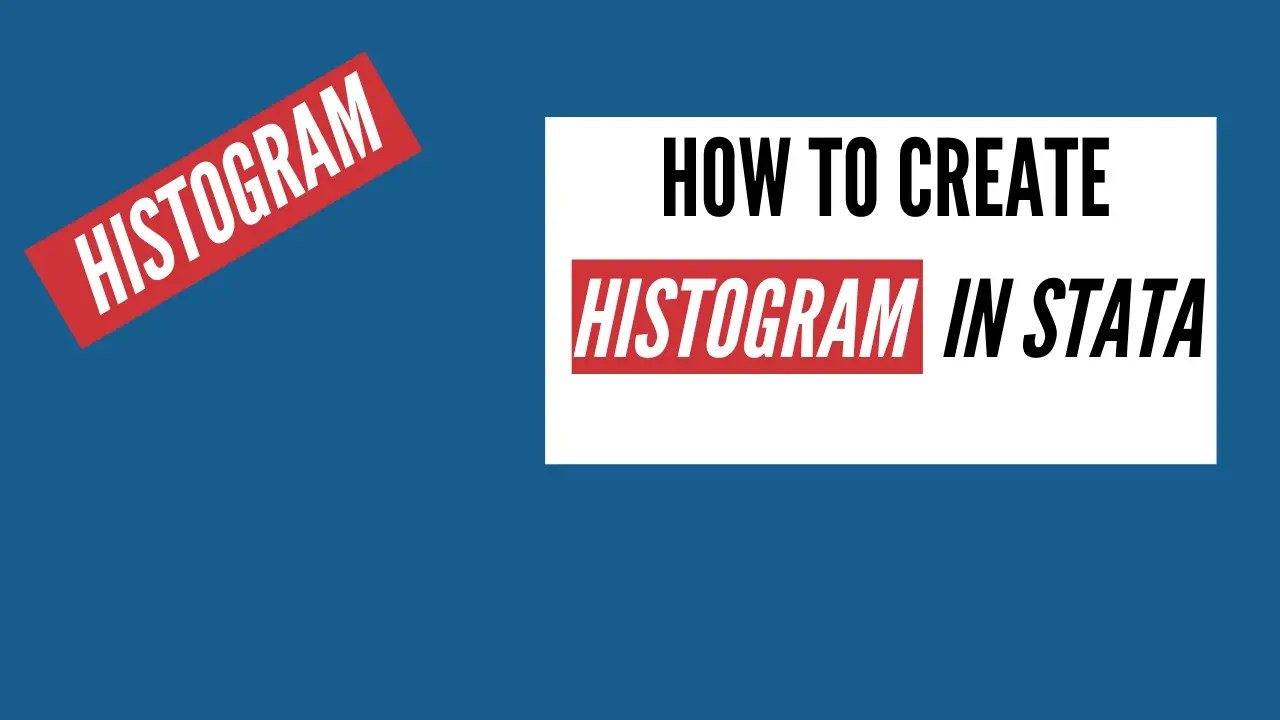# Stata Graphs

## Scatter plots in Stata

Scatter plot shows the relationship between dependent and independent variables and can be helpful in analyzing the data visually. In Stata, scatter plots can be generated to visualize this relationship and understand the pattern of graphs. This pattern can predict the correlation that exists between two variables and can even tell us whether this relationship …## How to Create A Histogram in Stata

Histograms are a common way of graphically representing the frequency distribution of data. In this article we are going to learn how to create Histogram in Stata Let’s load one of Stata’s inbuilt datasets to see how histograms are created. Go to File -> Example Datasets -> “Example Datasets Installed With Stata”. Click on the …